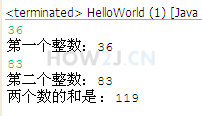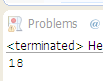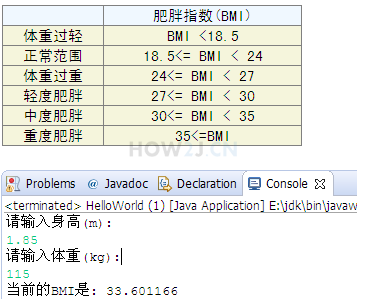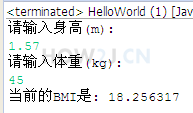+ - * / %

++ --13分21秒

## 基本算数操作符

+ - * /

public class HelloWorld { public static void main(String[] args) { int i = 10; int j = 5; int a = i+j; int b = i - j; int c = i*j; int d = i /j; } }
```public class HelloWorld {
public static void main(String[] args) {
int i = 10;
int j = 5;
int a = i+j;
int b = i - j;
int c = i*j;
int d = i /j;
}
}
```

## 练习-求和

姿势不对,事倍功半! 点击查看做练习的正确姿势## 答案-求和2分2秒 本视频采用html5方式播放，如无法正常播放，请将浏览器升级至最新版本，推荐火狐，chrome，360浏览器。 如果装有迅雷，播放视频呈现直接下载状态，请调整 迅雷系统设置-基本设置-启动-监视全部浏览器 (去掉这个选项)。 chrome 的 视频下载插件会影响播放，如 IDM 等，请关闭或者切换其他浏览器import java.util.Scanner; public class HelloWorld { public static void main(String[] args) { Scanner s = new Scanner(System.in); int a = s.nextInt(); System.out.println("第一个整数："+a); int b = s.nextInt(); System.out.println("第二个整数："+b); int c = a+b; System.out.println("两个数的和是 ：" + c); } }
```import java.util.Scanner;

public class HelloWorld {
public static void main(String[] args) {
Scanner s = new Scanner(System.in);
int a = s.nextInt();
System.out.println("第一个整数："+a);
int b = s.nextInt();
System.out.println("第二个整数："+b);
int c = a+b;
System.out.println("两个数的和是 ：" + c);
}
}
```

## 任意运算单元的长度超过int

int a = 5;
long b = 6;
a+b -> 结果类型是long
public class HelloWorld { public static void main(String[] args) { int a = 5; long b = 6; int c = (int) (a+b); //a+b的运算结果是long型，所以要进行强制转换 long d = a+b; } }
```public class HelloWorld {
public static void main(String[] args) {

int a = 5;
long b = 6;
int c = (int) (a+b); //a+b的运算结果是long型，所以要进行强制转换
long d = a+b;

}
}
```

## 任意运算单元的长度小于int

byte a = 1;
byte b= 2;
a+b -> int 类型
public class HelloWorld { public static void main(String[] args) { byte a = 1; byte b= 2; byte c = (byte) (a+b); //虽然a b都是byte类型，但是运算结果是int类型，需要进行强制转换 int d = a+b; } }
```public class HelloWorld {
public static void main(String[] args) {
byte a = 1;
byte b= 2;
byte c = (byte) (a+b); //虽然a b都是byte类型，但是运算结果是int类型，需要进行强制转换
int d = a+b;
}
}
```

## %取模

% 取余数，又叫取模
5除以2，余1
public class HelloWorld { public static void main(String[] args) { int i = 5; int j = 2; System.out.println(i%j); //输出为1 } }
```public class HelloWorld {
public static void main(String[] args) {

int i = 5;
int j = 2;
System.out.println(i%j); //输出为1
}
}
```

## 自增 自减

++
--

public class HelloWorld { public static void main(String[] args) { int i = 5; i++; System.out.println(i);//输出为6 } }
```public class HelloWorld {
public static void main(String[] args) {

int i = 5;
i++;
System.out.println(i);//输出为6

}
}
```

## 自增 自减操作符置前以及置后的区别

int i = 5;
i++; 先取值，再运算
++i; 先运算，再取值
public class HelloWorld { public static void main(String[] args) { int i = 5; System.out.println(i++); //输出5 System.out.println(i); //输出6 int j = 5; System.out.println(++j); //输出6 System.out.println(j); //输出6 } }
```public class HelloWorld {
public static void main(String[] args) {
int i = 5;
System.out.println(i++); //输出5
System.out.println(i);   //输出6

int j = 5;
System.out.println(++j); //输出6
System.out.println(j);	 //输出6
}
}
```

## 练习-自增

姿势不对,事倍功半! 点击查看做练习的正确姿势

int i = 1;
int j = ++i + i++ + ++i + ++i + i++;

## 答案-自增2分26秒 本视频采用html5方式播放，如无法正常播放，请将浏览器升级至最新版本，推荐火狐，chrome，360浏览器。 如果装有迅雷，播放视频呈现直接下载状态，请调整 迅雷系统设置-基本设置-启动-监视全部浏览器 (去掉这个选项)。 chrome 的 视频下载插件会影响播放，如 IDM 等，请关闭或者切换其他浏览器public class HelloWorld { public static void main(String[] args) { int i = 1; int j = ++i + i++ + ++i + ++i + i++; //i值 2 3 4 5 6 //取值 2 2 4 5 5 System.out.println(j); } }
```public class HelloWorld {
public static void main(String[] args) {
int i = 1;
int j = ++i + i++ + ++i + ++i + i++;
//i值        2     3     4     5     6
//取值      2     2     4     5     5
System.out.println(j);
}
}
```

## 练习-BMI

姿势不对,事倍功半! 点击查看做练习的正确姿势

BMI的计算公式是 体重(kg) / (身高*身高)

72 / (1.69*1.69) = ?## 答案-BMI4分19秒 本视频采用html5方式播放，如无法正常播放，请将浏览器升级至最新版本，推荐火狐，chrome，360浏览器。 如果装有迅雷，播放视频呈现直接下载状态，请调整 迅雷系统设置-基本设置-启动-监视全部浏览器 (去掉这个选项)。 chrome 的 视频下载插件会影响播放，如 IDM 等，请关闭或者切换其他浏览器import java.util.Scanner; public class HelloWorld { public static void main(String[] args) { Scanner s = new Scanner(System.in); System.out.println("请输入身高(m):"); float height = s.nextFloat(); System.out.println("请输入体重(kg):"); float weight = s.nextFloat(); float BMI = weight/ (height*height); System.out.println("当前的BMI是: " + BMI); } }
```import java.util.Scanner;

public class HelloWorld {
public static void main(String[] args) {
Scanner s = new Scanner(System.in);
System.out.println("请输入身高(m):");
float height = s.nextFloat();
System.out.println("请输入体重(kg):");
float weight = s.nextFloat();
float BMI = weight/ (height*height);
System.out.println("当前的BMI是: " + BMI);
}
}
```

HOW2J公众号，关注后实时获知最新的教程和优惠活动，谢谢。2021-10-05 这样写好像也可以哦，没用到long应该也没有问题吧？
 晚星 这样也可以吗，不用long，用double类型，因为老师讲这个类型用的比较多 ```Scanner i=new Scanner(System.in); System.out.println("请输入你的身高(m)："); double s=i.nextDouble(); System.out.println("请输入你的体重(kg)："); double t=i.nextDouble(); double b=t/(s*s); if(b<18.5) { System.out.println("体重过轻"); }else if(18.5<=b&&b<24) { System.out.println("正常范围"); }else if(24<=b&&b<27){ System.out.println("体重过胖"); }else if(27<=b&&b<30) { System.out.println("轻度肥胖"); }else if(30<=b&&b<35) { System.out.println("中度肥胖"); }else if(345<=b) { System.out.println("重度肥胖"); }else { System.out.println("别瞎鸡巴输入数据"); } System.out.println("当前的BIM是"+b); }```

4 个答案

jonty99

package lian; import java.util.Scanner; public class shuru { public static void main(String[] args){ System.out.println("请输入体重(kg)："); Scanner scanner = new Scanner(System.in); double weight = scanner.nextDouble(); System.out.println("请输入身高(m)："); //Scanner scanner = new Scanner(System.in); double height = scanner.nextDouble(); double BMI=weight/(height*height); System.out.println(BMI); }

c128ve980

package How2; import java.util.Scanner; public class ScannerTest { public static void main(String[] args) { System.out.println("请输入体重(kg)："); Scanner scanner = new Scanner(System.in); double weight = scanner.nextDouble(); System.out.println("请输入身高(m)："); double height = scanner.nextDouble(); double BMI = weight/(height*height); System.out.println(BMI); } }
```请输入体重(kg)：
85

1.75
27.755102040816325```

2021-09-16 强制转换时，时输入了什么快捷键呀？一下就变换了
 wong11 关于 JAVA 基础-操作符-算数操作符 的提问 在5:41的时候，是输入了什么快捷键进行的强制转换呀？ ```int i = 5; long j =61; int x = i+j;//需要转换，选中后输入的是什么快捷键```

1 个答案

jonty99

package lian; import java.util.Scanner; public class shuru { public static void main(String[] args){ System.out.println("请输入体重(kg)："); Scanner scanner = new Scanner(System.in); double weight = scanner.nextDouble(); System.out.println("请输入身高(m)："); //Scanner scanner = new Scanner(System.in); double height = scanner.nextDouble(); double BMI=weight/(height*height); System.out.println(BMI); }

2021-08-28 BMI练习
2021-07-23 为什么scanner s的s会报错
2021-07-03 计算BMI的小程序